Here we want to quickly explore some standard analyses for analog data (e.g. MUA or LFP measurements), and how to do these in Syncopy. Explorative coding is best done interactively by using e.g. Jupyter or IPython. Note that for plotting also matplotlib has to be installed.

Note

Installation of Syncopy itself is covered in here.

## Preparations¶

To start with a clean slate, let’s construct a synthetic dataset consisting of a damped harmonic and additive white noise:

```import numpy as np
import syncopy as spy

nTrials = 50
nSamples = 1000
nChannels = 2
samplerate = 500   # in Hz

# the sampling times vector needed for construction
tvec = np.arange(nSamples) * 1 / samplerate
# the 30Hz harmonic
harm = np.cos(2 * np.pi * 30 * tvec)
# dampening down to 10% of the original amplitude
dampening = np.linspace(1, 0.1, nSamples)
signal = dampening * harm

# collect trials
trials = []
for _ in range(nTrials):

trial = np.random.randn(nSamples, nChannels)
# now add the damped harmonic on the 1st channel
trial[:, 0] += signal
trials.append(trial)

# instantiate Syncopy data object
data = spy.AnalogData(trials, samplerate=samplerate)
```

With this we have a dataset of type `AnalogData`, which is intended for holding time-series data like electrophys. measurements. Let’s have a look at a small snippet of the 1st trial:

```data.singlepanelplot(trials=0, toilim=[0, 0.5])
```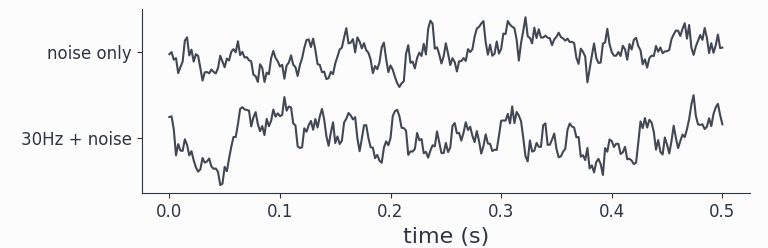By construction, we made the (white) noise of the same strength as the signal, hence by eye the oscillations present in `channel1` are hardly visible.

To recap: we have generated a synthetic dataset white noise on both channels, and `channel1` additionally carries the damped harmonic signal.

Hint

Further details about artificial data generation can be found at the Synthetic Data section.

## Data Object Inspection¶

We can get some basic information about any Syncopy dataset by just typing its name in an interpreter:

```data
```

which gives nicely formatted output:

```Syncopy AnalogData object with fields

cfg : dictionary with keys ''
channel :  element <class 'numpy.ndarray'>
container : None
data : 50 trials of length 1000.0 defined on [50000 x 3] float64 Dataset of size 1.14 MB
dimord : time by channel
filename : /xxx/xxx/.spy/spy_910e_572582c9.analog
mode : r+
sampleinfo : [50 x 2] element <class 'numpy.ndarray'>
samplerate : 500.0
tag : None
time : 50 element list
trialinfo : [50 x 0] element <class 'numpy.ndarray'>
trials : 50 element iterable

Use `.log` to see object history
```

So we see that we indeed got 50 trials with 2 channels and 1000 samples each. Note that Syncopy per default stores and writes all data on disk, as this allows for seamless processing of larger than memory datasets. The exact location and filename of a dataset in question is listed at the `filename` field. The standard location is the `.spy` directory created automatically in the user’s home directory. To change this and for more details please see Setting Up Your Python Environment.

Hint

You can access each of the shown meta-information fields separately using standard Python attribute access, e.g. `data.filename` or `data.samplerate`.

## Time-Frequency Analysis¶

Syncopy groups analysis functionality into meta-functions, which in turn have various parameters selecting and controlling specific methods. In the case of spectral analysis the function to use is `freqanalysis()`.

Here we quickly want to showcase two important methods for (time-)frequency analysis: (multi-tapered) FFT and Wavelet analysis.

### Multitapered Fourier Analysis¶

Multitaper methods allow for frequency smoothing of Fourier spectra. Syncopy implements the standard Slepian/DPSS tapers and provides a convenient parameter, the taper smoothing frequency `tapsmofrq` to control the amount of spectral smoothing in Hz. To perform a multi-tapered Fourier analysis with 3Hz spectral smoothing, we simply do:

```fft_spectra = spy.freqanalysis(data, method='mtmfft', foilim=[0, 50], tapsmofrq=2)
```

The parameter `foilim` controls the frequencies of interest limits, so in this case we are interested in the range 0-50Hz. Starting the computation interactively will show additional information:

```Syncopy <validate_taper> INFO: Using 3 taper(s) for multi-tapering
```

informing us, that for this dataset a spectral smoothing of 2Hz required 3 Slepian tapers.

The resulting new dataset `fft_spectra` is of type `syncopy.SpectralData`, which is the general datatype storing the results of a time-frequency analysis.

Hint

Try typing `fft_spectra.log` into your interpreter and have a look at Trace Your Steps: Data Logs to learn more about Syncopy’s logging features

To quickly have something for the eye we can plot the power spectrum of a single trial using the generic `syncopy.singlepanelplot()`:

```fft_spectra.singlepanelplot(trials=3)
```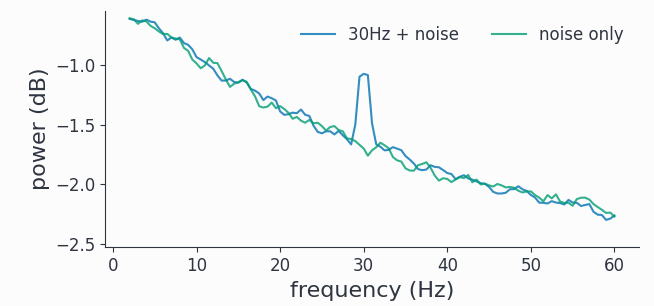We clearly see a smoothed spectral peak at 30Hz, channel 2 just contains the flat white noise floor. Comparing with the signals plotted in the time domain above, we see the power of the frequency representation of an oscillatory signal.

The related short time Fourier transform can be computed via `method='mtmconvol'`, see `freqanalysis()` for more details and examples.

### Wavelet Analysis¶

Wavelet Analysis, especially with Morlet Wavelets, is a well established method for time-frequency analysis. For each frequency of interest (`foi`), a Wavelet function gets convolved with the signal yielding a time dependent cross-correlation. By (densely) scanning a range of frequencies, a continuous time-frequency representation of the original signal can be generated.

In Syncopy we can compute the Wavelet transform by calling `freqanalysis()` with the `method='wavelet'` argument:

```# define frequencies to scan
fois = np.arange(10, 50, step=2) # 2Hz stepping
wav_spectra = spy.freqanalysis(data,
method='wavelet',
foi=fois,
parallel=True,
keeptrials=False)
```

Here we used two additional parameters supported by every Syncopy analysis method:

• `parallel=True` invokes Syncopy’s parallel processing engine

• `keeptrials=False` triggers trial averaging

Hint

If parallel processing is unavailable, have a look at Installing parallel processing engine ACME

To quickly inspect the results for each channel we can use:

```wav_spectra.multipanelplot()
```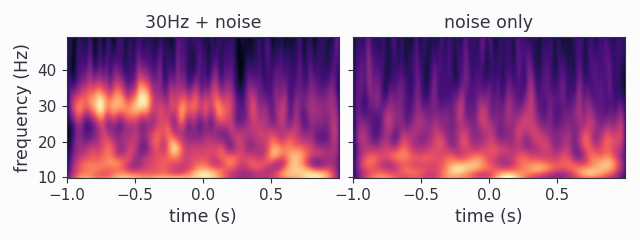Again, we see a strong 30Hz signal in the 1st channel, and channel 2 is devoid of any rhythms. However, in contrast to the `method=mtmfft` call, now we also get information along the time axis. The dampening of the harmonic over time in channel 1 is clearly visible.

An improved method, the superlet transform, providing super-resolution time-frequency representations can be computed via `method='superlet'`, see `freqanalysis()` for more details.

## Connectivity Analysis¶

Having time-frequency results for individual channels is useful, however we hardly learn anything about functional relationships between these different units. Even if two channels have a spectral peak at say 100Hz, we don’t know if these signals are actually connected. Syncopy offers various distinct methods to elucidate such putative connections via the `connectivityanalysis()` meta-function: coherence, cross-correlation and Granger-Geweke causality.

### Setup¶

To have a synthetic albeit meaningful dataset to illustrate the different methodologies we start by simulating two coupled autoregressive processes of order 2:

```import numpy as np
import syncopy as spy
from syncopy.tests import synth_data

nTrials = 50
nSamples = 1500
trls = []
# 2x2 Adjacency matrix to define coupling
# coupling 0 -> 1

for _ in range(nTrials):

trls.append(trl)

data = spy.AnalogData(trls, samplerate=500)
spec = spy.freqanalysis(data, tapsmofrq=3, keeptrials=False)
```

We also right away calculated the respective power spectra `spec`. We can quickly have a look at a snippet of the generated signals:

```data.singlepanelplot(trials=0, toilim=[0, 0.5])
```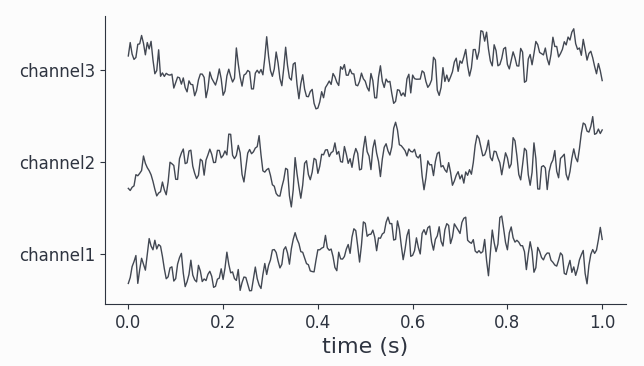Both channels show visible oscillations as is confirmed by looking at the power spectra:

```spec.singlepanelplot()
```As expected for the stochastic AR(2) model, we have a fairly broad spectral peak at around 100Hz.

### Coherence¶

One way to check for relationships between different oscillating channels is to calculate the pairwise coherence measure. It can be roughly understood as a frequency dependent correlation. Let’s do this for our coupled AR(2) signals:

```coherence = spy.connectivityanalysis(data, method='coh', tapsmofrq=3)
```

The result is of type `spy.CrossSpectralData`, the standard datatype for all connectivity measures. It contains the results for all `nChannels x nChannels` possible combinations. Let’s pick the two available channel combinations and plot the results:

```coherence.singlepanelplot(channel_i='channel1', channel_j='channel2')
coherence.singlepanelplot(channel_i='channel2', channel_j='channel1')
```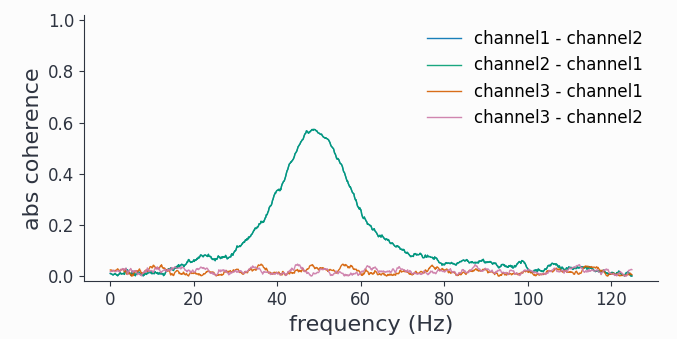As coherence is a symmetric measure, we have the same graph for both channel combinations, showing high coherence around 100Hz.

Note

The plotting for `CrossSpectralData` object works a bit differently, as the user here has to provide one channel combination for each plot with the keywords `channel_i` and `channel_j`.

### Cross-Correlation¶

Coherence is a spectral measure for correlation, the corresponding time-domain measure is the well known cross-correlation. In Syncopy we can get the cross-correlation between all channel pairs with:

```corr = spy.connectivityanalysis(data, method='corr', keeptrials=True)
```

As this also is a symmetric measure, let’s just look at the only channel combination however this time for two different trials:

```corr.singlepanelplot(channel_i=0, channel_j=1, trials=0)
corr.singlepanelplot(channel_i=0, channel_j=1, trials=1)
```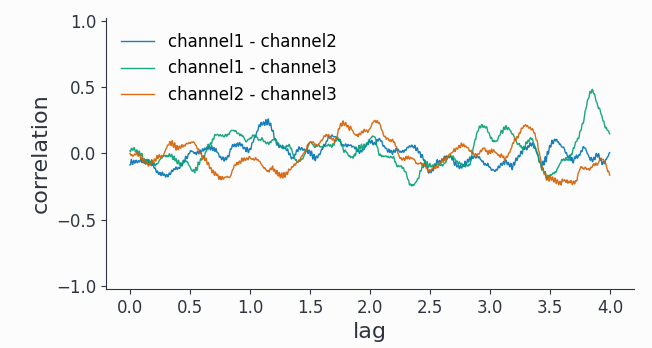We see that there are persistent correlations also for longer lags.

### Granger Causality¶

To reveal directionality, or causality, between different channels Syncopy offers the Granger-Geweke algorithm for non-parametric Granger causality in the spectral domain:

```granger = spy.connectivityanalysis(data, method='granger', tapsmofrq=2)
```

Now we want to see differential causality, so we plot both channel combinations:

```granger.singlepanelplot(channel_i=0, channel_j=1)
granger.singlepanelplot(channel_i=1, channel_j=0)
```

This reveals the coupling structure we put into this synthetic data set: `channel1` influences `channel2`, but in the other direction there is no interaction.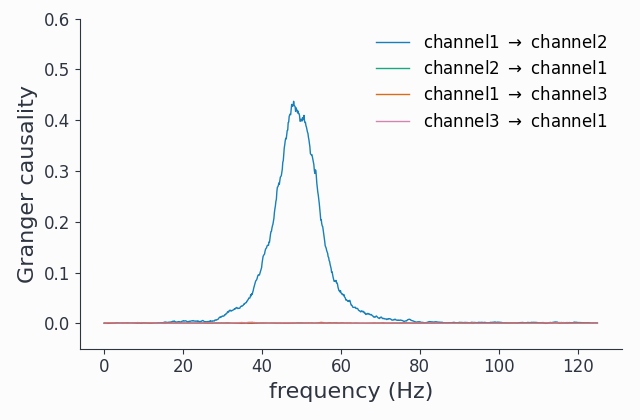Note

The `keeptrials` keyword is only valid for cross-correlations, as both Granger causality and coherence critically rely on trial averaging.